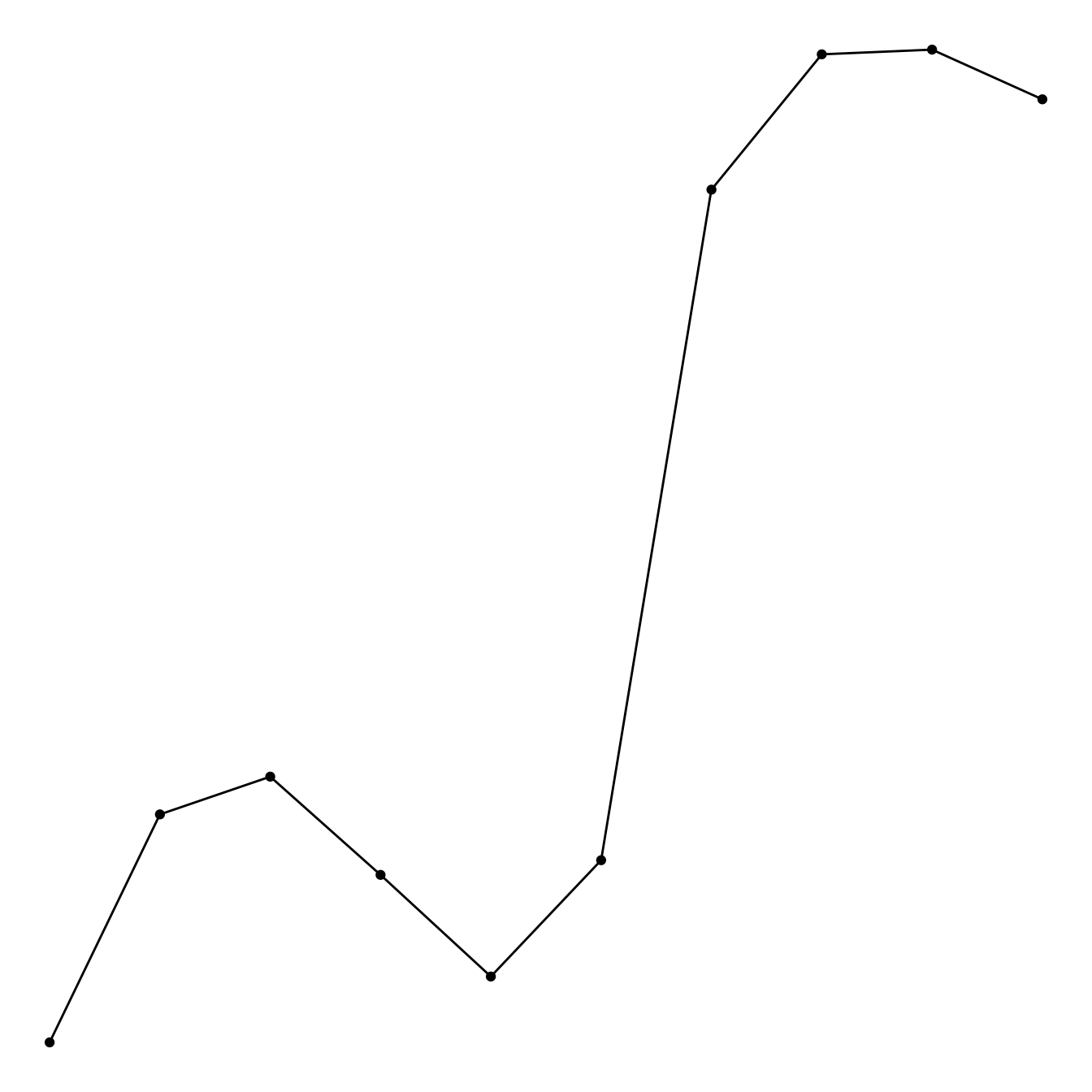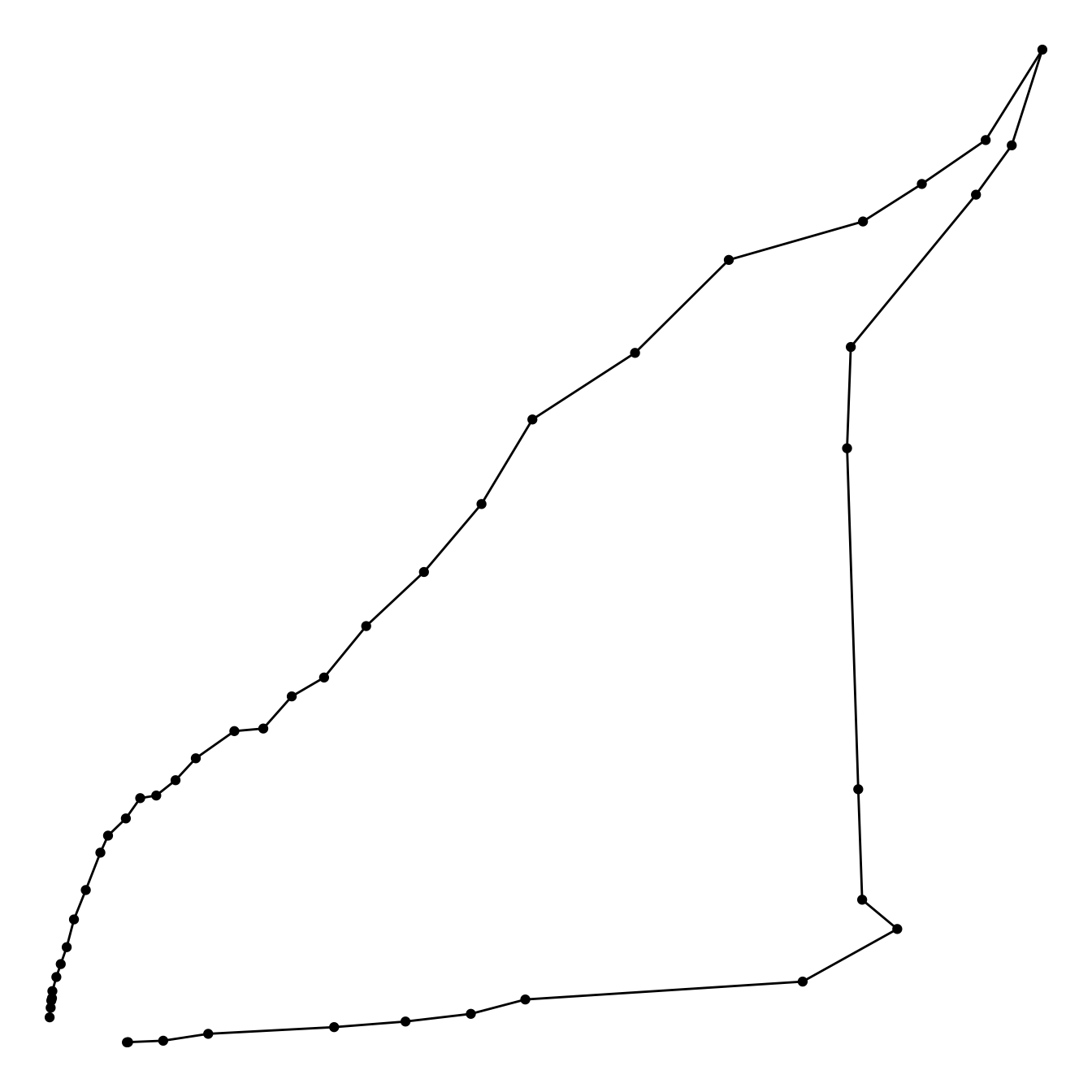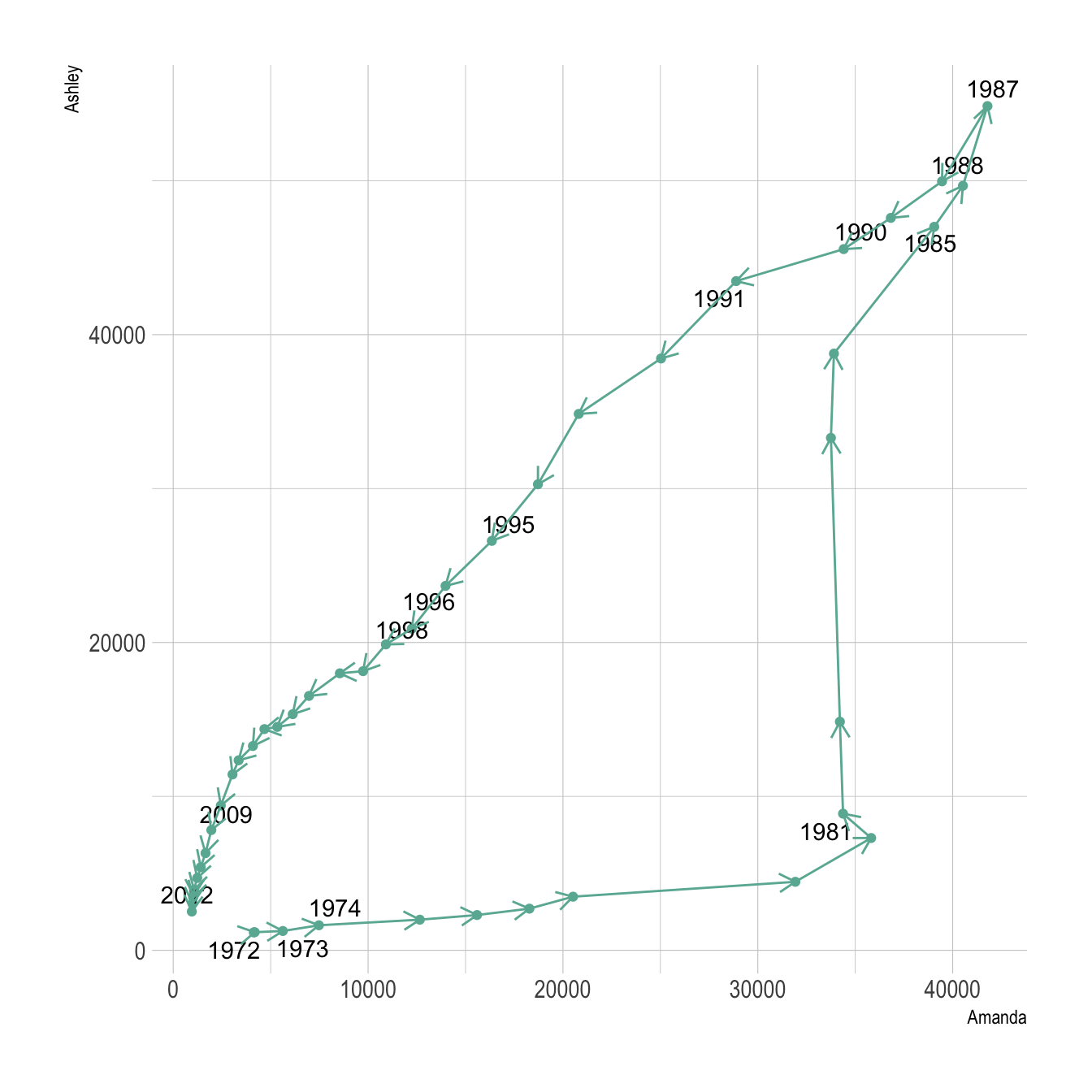# Connected scatterplot with R and ggplot2

This post explains how to build a basic connected scatterplot with R and ggplot2. It provides several reproducible examples with explanation and R code.

# Most basic connected scatterplot: `geom_point()` and `geom_line()`

A connected scatterplot is basically a hybrid between a scatterplot and a line plot. Thus, you just have to add a `geom_point()` on top of the `geom_line()` to build it.``````# Libraries
library(ggplot2)
library(dplyr)

# Load dataset from github
data <- read.table("https://raw.githubusercontent.com/holtzy/data_to_viz/master/Example_dataset/3_TwoNumOrdered.csv", header=T)
data\$date <- as.Date(data\$date)

# Plot
data %>%
tail(10) %>%
ggplot( aes(x=date, y=value)) +
geom_line() +
geom_point()``````

# Customize the connected scatterplot

Custom the general theme with the `theme_ipsum()` function of the `hrbrthemes` package. Add a title with `ggtitle()`. Custom circle and line with arguments like `shape`, `size`, `color` and more.``````# Libraries
library(ggplot2)
library(dplyr)
library(hrbrthemes)

# Load dataset from github
data <- read.table("https://raw.githubusercontent.com/holtzy/data_to_viz/master/Example_dataset/3_TwoNumOrdered.csv", header=T)
data\$date <- as.Date(data\$date)

# Plot
data %>%
tail(10) %>%
ggplot( aes(x=date, y=value)) +
geom_line( color="grey") +
geom_point(shape=21, color="black", fill="#69b3a2", size=6) +
theme_ipsum() +
ggtitle("Evolution of bitcoin price")``````

# Connected scatterplot to show an evolution

The connected scatterplot can also be a powerfull technique to tell a story about the evolution of 2 variables. Let???s consider a dataset composed of 3 columns:

• Year
• Number of baby born called Amanda this year
• Number of baby born called Ashley

The scatterplot beside allows to understand the evolution of these 2 names. Note that the code is pretty different in this case. `geom_segment()` is used of `geom_line()`. This is because `geom_line()` automatically sort data points depending on their X position to link them.``````# Libraries
library(ggplot2)
library(dplyr)
library(babynames)
library(ggrepel)
library(tidyr)

# data
data <- babynames %>%
filter(name %in% c("Ashley", "Amanda")) %>%
filter(sex=="F") %>%
filter(year>1970) %>%
select(year, name, n) %>%
spread(key = name, value=n, -1)

# plot
data %>%
ggplot(aes(x=Amanda, y=Ashley, label=year)) +
geom_point() +
geom_segment(aes(
xend=c(tail(Amanda, n=-1), NA),
yend=c(tail(Ashley, n=-1), NA)
)
) ``````

It makes sense to add arrows and labels to guide the reader in the chart:``````# Libraries
library(ggplot2)
library(dplyr)
library(babynames)
library(ggrepel)
library(tidyr)

# data
data <- babynames %>%
filter(name %in% c("Ashley", "Amanda")) %>%
filter(sex=="F") %>%
filter(year>1970) %>%
select(year, name, n) %>%
spread(key = name, value=n, -1)

# Select a few date to label the chart
tmp_date <- data %>% sample_frac(0.3)

# plot
data %>%
ggplot(aes(x=Amanda, y=Ashley, label=year)) +
geom_point(color="#69b3a2") +
geom_text_repel(data=tmp_date) +
geom_segment(color="#69b3a2",
aes(
xend=c(tail(Amanda, n=-1), NA),
yend=c(tail(Ashley, n=-1), NA)
),
arrow=arrow(length=unit(0.3,"cm"))
) +
theme_ipsum()``````

Related chart types

## Contact

This document is a work by Yan Holtz. Any feedback is highly encouraged. You can fill an issue on Github, drop me a message on Twitter, or send an email pasting yan.holtz.data with gmail.com.